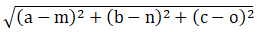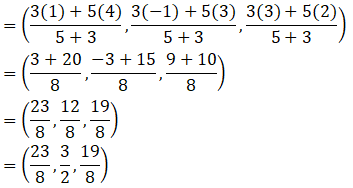The vertices of the triangle are A(5, 4, 6), B(1, -1, 3) and C(4, 3, 2). The internal bisector of angle A meets BC at D. Find the coordinates of D and the length AD.

Asked by Sakshi | 1 year ago |  36

##### Solution :-

Given:

The vertices of the triangle are A (5, 4, 6), B (1, -1, 3) and C (4, 3, 2).

By using the formulas let us find the coordinates of D and the length of AD

The distance between any two points (a, b, c) and (m, n, o) is given by,The distance between A (5, 4, 6), B (1, -1, 3) is AB

$$\sqrt{(5-2)^2+4-(-1)^2+(6-3)^2}$$

$$\sqrt{16+25+9}=5\sqrt{2}$$

The distance between A (5, 4, 6), C (4, 3, 2) is AC

$$\sqrt{(5-4)^2+(4-3)^2+(6-2)^2}$$

$$\sqrt{1+1+16}=3\sqrt{2}$$

AB : AC = 5:3

BD: DC = 5:3

So, m = 5 and n = 3

B(1, -1, 3) and C(4, 3, 2)

Coordinates of D using section formula:The coordinates of D are $$( \dfrac{23}{8},\dfrac{3}{2},\dfrac{19}{8})$$

Answered by Aaryan | 1 year ago

### Related Questions

#### A(1, 2, 3), B(0, 4, 1), C(-1, -1, -3) are the vertices of a triangle ABC. Find the point in which the bisector of the

A(1, 2, 3), B(0, 4, 1), C(-1, -1, -3) are the vertices of a triangle ABC. Find the point in which the bisector of the angle ∠BAC meets BC.

#### The mid-points of the sides of a triangle ABC are given by (-2, 3, 5), (4, -1, 7) and (6, 5, 3). Find the coordinates

The mid-points of the sides of a triangle ABC are given by (-2, 3, 5), (4, -1, 7) and (6, 5, 3). Find the coordinates of A, B and C.

#### If the points A(3, 2, -4), B(9, 8, -10) and C(5, 4, -6) are collinear, find the ratio in which C divided AB.

If the points A(3, 2, -4), B(9, 8, -10) and C(5, 4, -6) are collinear, find the ratio in which C divided AB.